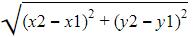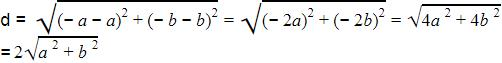Courses

# Practice Test: Coordinate Geometry

## 25 Questions MCQ Test Mathematics (Maths) Class 10 | Practice Test: Coordinate Geometry

Description
This mock test of Practice Test: Coordinate Geometry for Class 10 helps you for every Class 10 entrance exam. This contains 25 Multiple Choice Questions for Class 10 Practice Test: Coordinate Geometry (mcq) to study with solutions a complete question bank. The solved questions answers in this Practice Test: Coordinate Geometry quiz give you a good mix of easy questions and tough questions. Class 10 students definitely take this Practice Test: Coordinate Geometry exercise for a better result in the exam. You can find other Practice Test: Coordinate Geometry extra questions, long questions & short questions for Class 10 on EduRev as well by searching above.
QUESTION: 1

### The distance between the points (a, b) and (– a, – b) is :

Solution:

We have distance formula as d =Where x1=a,y1=b,x2=-a,y2=-bQUESTION: 2

### The distance between points (a + b, b + c) and (a – b, c – b) is :

Solution: Distance between two point is
root[{(a+b)-(a-b)}^2] + [{(b+c)-(c-b)}^2]
=root [{(a+b-a+b)^2}] + [((b+c-c+b)}^2]
=root[(2b)^2 + (2b)^2]
=root[4b^2 +4b^2]
=root[8b^2]
=2.root(2)b
QUESTION: 3

### The distance between A (1, 3) and B (x, 7) is 5. The value of x > 0 is :

Solution:
QUESTION: 4

The distance between the points (a cos 20° + b sin 20°, 0) and (0, a sin 20° – b cos 20°) is :

Solution:
QUESTION: 5

Mid-point of the line-segment joining the points (– 5, 4) and (9, – 8) is :

Solution:
QUESTION: 6

The co-ordinates of the points which divides the join of (– 2, – 2) and (– 5, 7) in the ratio 2 : 1 is :

Solution:
QUESTION: 7

The co-ordinates of the point on x-axis which is equidistant from the points (5, 4) and (– 2, 3) are :

Solution:

Let the point on x-axis be (a,0)
Since PA = PB  PA2 = PB2
(a + 2)2 + (0 - 5)2 = (a - 2)2 + (0 + 3)2
(a + 2)2 - (a - 2)2 = 9 - 25 = -16
8a = -16  a = -2.
The required point is (-2,0).

QUESTION: 8

The co-ordinates of the point on y-axis which is equidistant from the points (3, 1) and (1, 5) are:

Solution:
QUESTION: 9

The coordinates of the centre of a circle are (– 6, 1.5). If the ends of a diameter are (– 3, y) and (x, – 2) then:

Solution:
QUESTION: 10

The points (– 2, 2), (8, – 2) and (– 4, – 3) are the vertices of a :

Solution:
QUESTION: 11

The points (1, 7), (4, 2), (– 1, 1) and (– 4, 4) are the vertices of a :

Solution:

Step-by-step explanation:

Given points are (1,7),(4,2),(-1,-1),(-4,4)

Let the points are A,B,C,D.

Distance formula = √(x₂-x₁)²+(y₂-y₁)²

AB = √(4-1)²+(2-7)²

= √9+25

= √34

BC = √(-1-4)²+(-1-2)²

= √25+9

=  √34

CD = √(-4-(-1))²+(4-(-1))²

= √9+25

=  √34

DA = √(1-(-4))²+(7-4)²

= √25+9

= √34

also diagonals AC and BD

AC = √(1+1)² + (7+1)² = √68

BD = √(4+4)² + (2-4)² = √68

We know that the all sides and both diagonals of the square are equal

So,from this we can say that these points are the vertices of a square.

QUESTION: 12

The line segment joining (2, – 3) and (5, 6) is divided by x-axis in the ratio:

Solution:
QUESTION: 13

The line segment joining the points (3, 5) and (– 4, 2) is divided by y-axis in the ratio:

Solution:
QUESTION: 14

If (3, 2), (4, k) and (5, 3) are collinear then k is equal to :

Solution:
QUESTION: 15

If the points (p, 0), (0, q) and (1, 1) are collinear then 1/p+1/q is equal to :

Solution:
QUESTION: 16

Two vertices of a triangle are (–2, – 3) and (4, –1) and centroid is at the origin. The coordinates of the third vertex of the triangle are :

Solution:
QUESTION: 17

A (5, 1), B(1, 5) and C (–3, –1) are the vertices of ?ABC. The length of its median AD is :

Solution:
QUESTION: 18

Three consecutive vertices of a parallelogram are (1, –2), (3, 6) and (5, 10). The coordinates of the fourth vertex are :

Solution:
QUESTION: 19

The vertices of a parallelogram are (3, –2), (4, 0), (6, –3) and (5, –5). The diagonals intersect at the point M. The coordinates of the point M are :

Solution:
QUESTION: 20

If two vertices of a parallelogram are (3, 2) and (–1, 0) and the diagonals intersect at (2, –5), then the other two vertices are :

Solution:
QUESTION: 21

The circumcentre of the triangle formed by the lines xy + 2x + 2y + 4 = 0 and x + y + 2 = 0 is :

Solution:
QUESTION: 22

The vertices of a triangle are (a, b – c), (b, c – a) and (c, a – b), then it's centroid lies on :

Solution:
QUESTION: 23

The points (1, 2), (3, 8) and (x, 20) are collinear if x =

Solution:
QUESTION: 24

For the triangle whose sides are along the lines x = 0, y = 0 and x/6+y/8 = 1, the incentre is :

Solution:
QUESTION: 25

For the triangle whose sides are along the lines y = 15, 3x – 4y = 0, 5x + 12y = 0, the incentre is :

Solution: